Two loudspeakers in a plane, 5.0 m apart, are playing the same frequency. If you stand 12.0 m in front of the plane of the speak- ers, cente

Question

Two loudspeakers in a plane, 5.0 m apart, are playing the same frequency. If you stand 12.0 m in front of the plane of the speak- ers, centered between them, you hear a sound of maximum in- tensity. As you walk parallel to the plane of the speakers, staying 12.0 m in front of them, you first hear a minimum of sound inten- sity when you are directly in front of one of the speakers. What is the frequency of the sound? Assume a sound speed of 340 m/s

in progress 0
6 months 2021-07-15T03:45:00+00:00 2 Answers 23 views 0

The frequency of the sound is 170 Hz

Explanation:

Traveling wave is given as;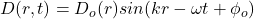where;

r is the distance from the source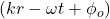is phase

Then phase difference is given as;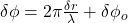The phase difference between the two speakers at maximum intensity;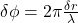where;

λ is the wavelength

Δr is difference in distance between the two speakers

Δr = r₂ – r₁ =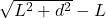Given;

distance between the two speakers, d = 5.0 m

distance to the plane of the speakers, L = 12.0 m

Δr =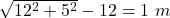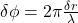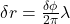, at minimum sound intensity, ΔФ = π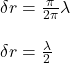λ = 2Δr

λ = 2 (1) = 2m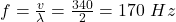Therefore, the frequency of the sound is 170 Hz

170 Hz

Explanation:

The path difference between two waves are given by:

Δ=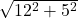– 12

Δ = 1 m

In order to hear minimum intensity, the path difference will be

Δ = λ/2

λ = 2Δ

Also, We know that

V= f λ

λ=V/f

Therefore,

340/f = 2 x 1

f= 170 Hz

Thus, the frequency of the sound is 170 Hz